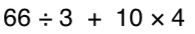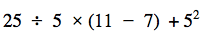Equations
Exponents
Order of Operations
Simplify Expressions
Fractions, %, and decimals
Worded Questions
200

3x + 7 = 37

10

200

What is the 2 in this expression? What is the 4?

24

2 - base number

4 - exponent or power

200

Simplify the following expression:62

200

-8(4x+2)

-32x-16

200

which is the largest, 25%, 0.27, or 2/5?

0.27

200

Balia knows that 6 cups of rice will feed 15 people.  How many cups of rice are needed to feed 135 people?

54 cups

300

-11k-1=65

-6

300

How can you rewrite the following in exponential form?

7 x 7 x 7 x 7 x 7

75

300

3 + 42 x (3 + 2)

83

300

-9(2x - 3)

-18x + 20

300

what is 6% written as a decimal?

0.06

300

Arrange from fastest to slowest:

Abel reads 50 pages in 45 minutes; Brian reads 90 pages in 75 minutes; Charlie reads 175 pages in 2 hours

Charlie, Brian, Abel

400

3(4g+15)-7=-10

-4

400

How can you rewrite 93 in factor form?

9 x 9 x 9

400

Use the order of operations:

3 x 4 + 2 x 6

24

400

18+ 5(3x - 7)

15x-17

400

write 3/5 as a percent

60%

400

A train in Japan can travel 813.5 miles in 5 hrs.  Find the unit rate in miles per hour

162.7 mi / hr

600

6y+11 = 8y-15

13

600

Write in factor form

8x 63

8x8x8x8x6x6x6

60045

600

-2 + -3 + 5

0

600

10 -3(6x - 10) + -4(x-7)

-22x+68

600

what is 3/20 written as a percent?

15%

600

Stacy's son ran 48 miles over the past two weeks. How many miles did he run each day? Round to the nearest tenth

3.4 miles per day

1000

6a-2(4a-5)=40

-15
1000

What is the value of:

23 + 32

17

100030
1000

-50 -(-15)

-35

1000

x + 10 - 6(x - 3x) + 4

13x + 14

1000

What is 0.34 written as a fraction in simplest form?

17/50

1000

Stacy's dog ate 21 pounds of food last week. If he is fed twice a day, how many pounds did he eat at each meal?

1.5

Click to zoom